# Geometry optimization: CO/Pd(100)¶

Version: 2015.1

In this tutorial you will learn how to do a geometry optimization using Fixed and Rigid constrains on the atoms. As an example, you will study adsorption of the CO molecule on a Pd(100) surface. You will use the ATK-DFT calculator engine and a standard GGA functional.

Specifically, you will:

1. optimize the bulk palladium crystal;
2. cleave the bulk to create the Pd(100) surface and relax it;
3. adsorb the CO molecule in the surface, and relax the system in two steps;
1. first apply Rigid constraints on atoms during an initial relaxation;
2. then apply Fixed constraints on the bottom Pd atoms in a final optimization.
4. lastly, relax the CO molecule and calculate the adsorption energy.Create a new project called “Pd100_CO” and open the Builder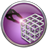.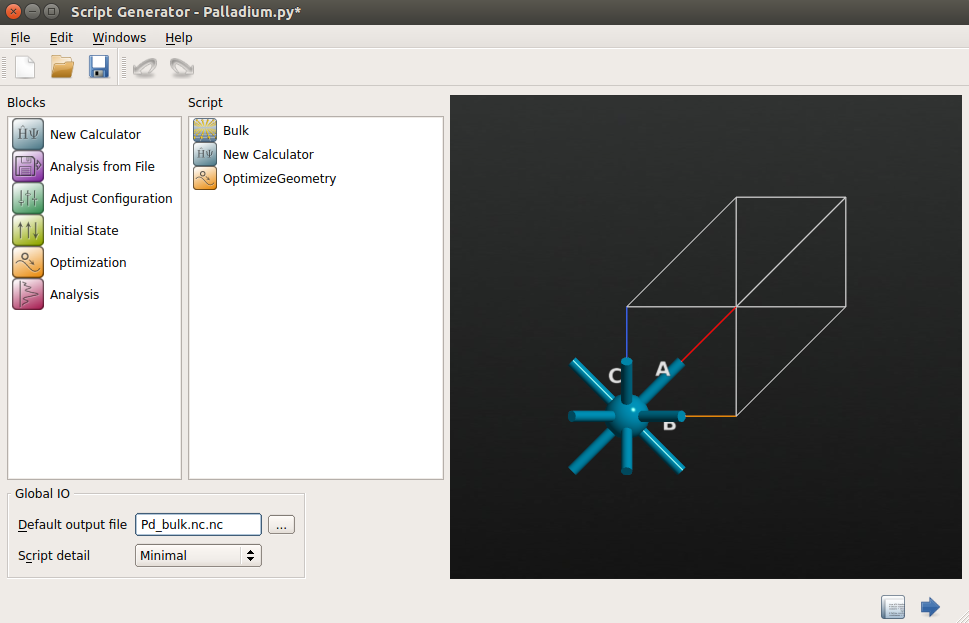Tip

It is usually a good idea to save the trajectory file. In case your geometry optimization is interrupted you can simply restart it from one of the latest images in the trajectory. If you would like to know more about how to restart a stopped calculation, check out the tutorial Restarting a stopped calculation.

Once the bulk relaxation is finished, the calculation output will appear on the LabFloor. The bulk configurations with IDs glD000 and glD002 are the initial and final structures, respectively, while the relaxation trajectory has ID glD001.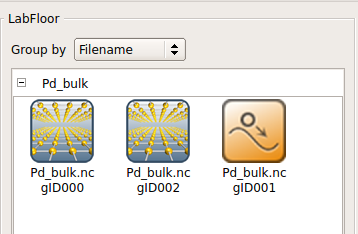You can drag and drop these objects to the Viewerto visualize them. In the Viewer, you can check the lattice constant by hovering the mouse on the cell boundaries. You could also check the lattice constant in the newly formed log file.

In the following, you should of course use the relaxed bulk structure, glD002.

## Build the Pd(100) surface and relax it¶

• Drag and drop the relaxed bulk configuration to the Builder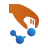.
• Open the Builders -‣ Surface (Cleave) plug-in.
• Keep the default Miller indices and click Next.
• Keep the default 1x1 surface lattice and click Next.
• Choose a Non-periodic and normal (slab) out-of-plane cell vector, 4 slab metal layers, and 10 Å and 5 Å for the top and bottom vacuums, respectively. Click Finish to build the surface.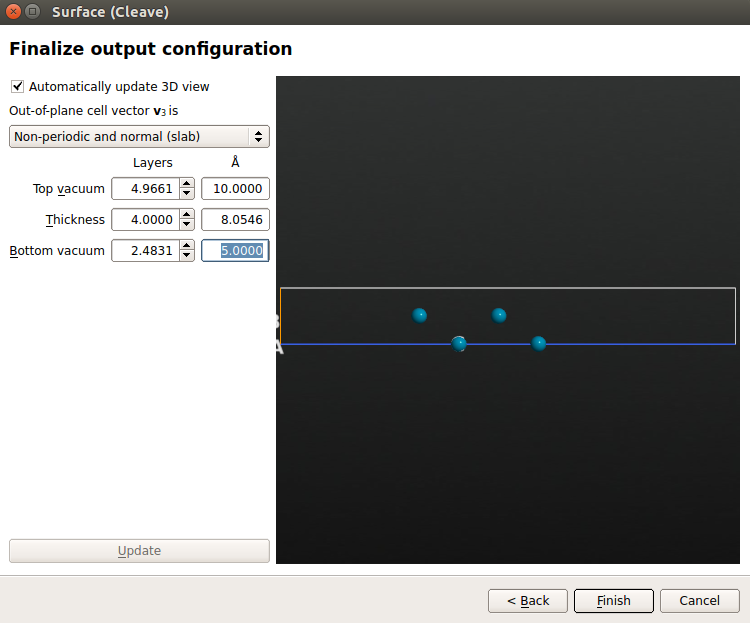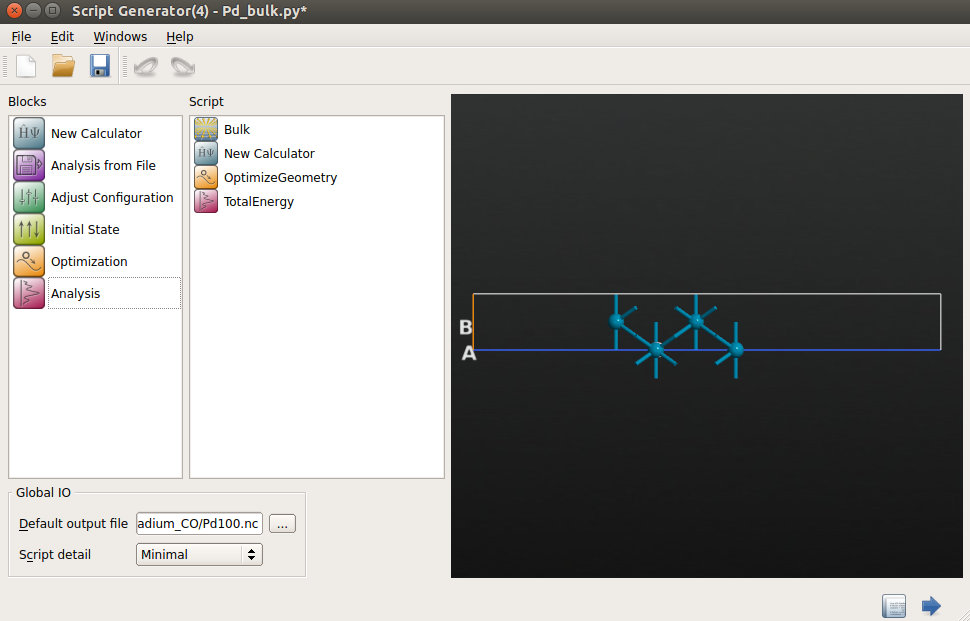• Choose the following set of calculator parameters:

• k-point sampling: 9x9x1 k-points;
• exchange-correlation: GGA-PBE;
• FFT2D Poisson solver with Neumann boundary conditions under the slab and Dirichlet boundary conditions above the slab: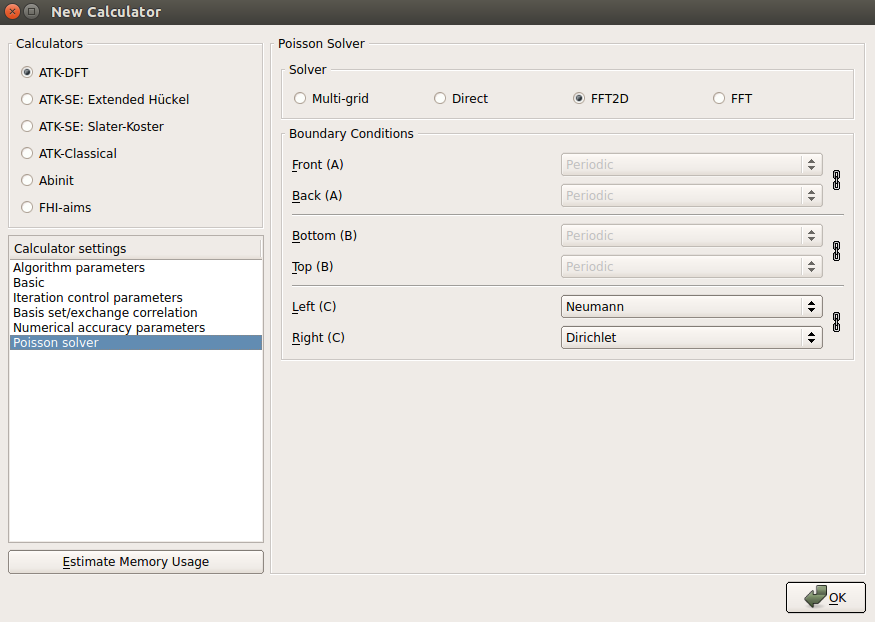Note

This particular set of boundary conditions along the C direction of the simulation cell is appropriate for slab calculations, where the vacuum above the slab could in principle extend to infinity.

• Open theOptimizeGeometry block and set it up for force relaxation:
• save the trajectory to Pd100_traj.hdf5;
• click Add Constraints to open the Constraints Editor;
• use the mouse to select the bottom two layers of the Pd(100) surface and click Add tag from selection. Those two atoms are now tagged “Selection 0”. Then choose the Fixed constraint for the “Selection 0” group.Once the Pd(100) surface has been geometry optimized, the results will appear on the LabFloor. You can again visualize the relaxation trajectory using the Viewer.

Tip

You can check the total energy of the relaxed Pd(100) surface by selecting the TotalEnergy object and clicking on the Text Representation plugin. The energy is also printed in the log file.

## Relax the CO/Pd(100) system¶

The next step is to add the CO molecule to the surface and relax the system. You should use the CO/Pd(100) structure available here: CO_Pd100.py. If needed, you can learn how to add a molecule to a surface from the tutorial Building molecule–surface systems: Benzene on Au(111).

As mentioned in the introduction, you will relax the CO/Pd(100) configuration in two steps:

1. use a Rigid constraint for CO and Fixed constrain for the surface, together with a relatively low electron density mesh cut-off, in order to get a quick idea of where the CO will be adsorbed;
2. the final relaxation step does not constrain the CO molecule and just fixes the bottom of the Pd(100) slab.

### Rigid relaxation¶

Transfer the provided CO/Pd(100) configuration (CO_Pd100.py) to the Script Generator and add the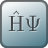New Calculator andOptimizeGeometry blocks. Enter Pd100_CO_rigid.hdf5 for the default output file name, and then edit the script:• Set up theOptimizeGeometry block similarly to the one used for Pd(100), but choose a slightly different set of constraints:
• select the Fixed constraint for all atoms in the metal slab;
• select the Rigid constraint for the CO molecule (add the C and O atoms to the group “Selection 1”).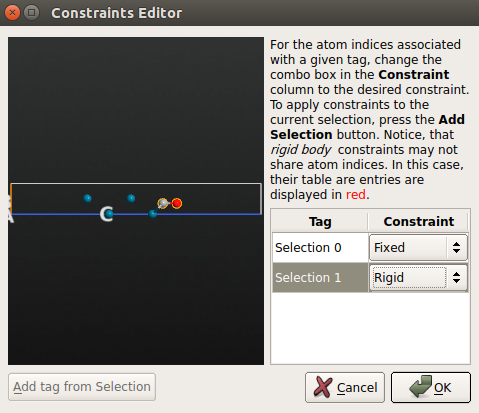Note

The Rigid constraint on a group of atoms causes those atoms to move like a rigid body during force optimization.

• Save the script as Pd100_CO_rigid.py and run it using the Job Manager. The simulation should take roughly 5 minutes if executed in serial. You can download the script here: Pd100_CO_rigid.py.

Important

If you take a look at the trajectory, you will see that the CO molecule has moved slightly away from its initial position, but the C–O bond length has not changed, and the molecule has not rotated. This is how the rigid constraint works.

### Final relaxation¶

You will now perform the final geometry optimization of the CO/Pd(100) structure, starting from the result of the previous step.• Save the script as Pd_CO.py and execute it using the Job Manager or in a Terminal. The calculations should take about 20 min in total, but can of course be quicker if run in parallel on more CPUs. The script is also available here: Pd100_CO.py.

Tip

You can actually use the Viewertool to monitor the progress of the geometry optimization while the calculation is running. The trajectory object is continuously updated as new relaxations steps are taken.

When the calculation has finished you should have a look at the full relaxation trajectory, and see how the CO molecule has moved from a position around the hollow adsorption site on the Pd(100) surface to a bridge site between two Pd surface atoms:## Relax the CO molecule¶

In order to calculate the CO adsorption energy on Pd(100) you also need to relax the isolated CO molecule. We can obtain it following the next steps:

• go to the Builder and add a new configuration using Add ‣ New Configuration;
• click the Molecular Builder tool and click Fragments ‣ Carbon monoxide in the panel that opens up;• next, click the hydrogen atom in the New Configuration Stash item to substitute it for the CO fragment, close the Molecular Builder window, and rename the Stash item to CO.Tip

For more details about how to use the Molecular Builder, you can check out this tutorial: Molecular builder.

Now you just need to relax the CO atomic coordinates and compute the total energy. Send the structure to the Script Generator and add theNew Calculator,OptimizeGeometry and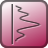TotalEnergy blocks. Enter CO.hdf5 for the default output file name, and then edit the blocks a bit:

Save the script as CO.py and run the calculation – it should be very fast. The script is also available for download: CO.py.

You will find that the C–O bond length relaxes to 1.143 Å, which matches experiments very well.

You are now ready to calculate the CO/Pd(100) adsorption energy at a full monolayer coverage, $$\Delta E$$, which is of course given by total energy differences:

$\Delta E = E_\mathrm{products} - E_\mathrm{reactants} = E_\mathrm{Pd(100)/CO} - E_\mathrm{Pd(100)} - E_\mathrm{CO}$

You can use the Text Representation plugin to see all three total energies and then calculate $$\Delta E$$, or you can use a script to do it: adsorption_energy.py.

The adsorption energy, calculated using PBE and the FHI pseudopotentials with a DZP basis set, is $$\Delta E$$ = –1.97 eV. However, this number is affected by the so-called basis set superposition error, so you should proceed to the next section to correct for this.

### Counterpoise correction¶

The basis set superposition error (BSSE) is due to the incompleteness of the LCAO basis set, and can have a significant impact on energy differences between different sub-systems. As explained in a tutorial (DFT-D and basis-set superposition error), the superposition of Pd and CO basis sets in the CO/Pd(100) system can give rise to an artificial lowering of the total energy because the Pd(100) and CO sub-systems can “borrow” basis functions from each other. The result is a too large adsorption energy.

The standard approach to neutralize the BSSE is to apply a so-called counterpoise correction. In QuantumATK, this is done using the counterpoiseCorrected.

You should therefore calculate the counterpoise (CP) corrected total energy for the CO/Pd(100) system in order to compute the CP corrected adsorption energy:

$\Delta E^\mathrm{CP} = E^\mathrm{CP}_\mathrm{Pd(100)/CO} - E_\mathrm{Pd(100)} - E_\mathrm{CO}$

Use the script shown below (can be downloadad here: Pd_CO_cp.py). The script reads in the previously relaxed CO/Pd(100) configuration and the calculator that was used, and creates a new calculator that applies the CP correction. The configuration is then relaxed and the total energy is calculated.

Run the script using the Job Manager or in a Terminal. It should perform 4 very small relaxation steps, and finish within 10 minutes. The CP correction will increase the total energy of the CO/Pd(100) system, resulting in an adsorption energy of $$\Delta E^\mathrm{CP}$$ = –1.68 eV.

  1 2 3 4 5 6 7 8 9 10 11 12 13 14 15 16 17 18 19 20 21 22 23 24 25 26 27 28 29 30 31 32 33 34 35 36 37 38 39 40 41 42 43 44 45 46 47 48 49 50 51 52 53 54 55 56 57 58 59 60 # ------------------------------------------------------------- # Bulk Configuration # ------------------------------------------------------------- bulk_configuration = nlread('Pd100_CO.nc', BulkConfiguration)[-1] # Add tags bulk_configuration.addTags('CO', [4, 5]) bulk_configuration.addTags('Pd', [0, 1, 2, 3]) bulk_configuration.addTags('Selection 0', [0, 1]) # ------------------------------------------------------------- # Calculator # ------------------------------------------------------------- # Get the calculator settings from non-BSSE calculation. calculator = bulk_configuration.calculator() basis_set = calculator.basisSet() exchange_correlation = calculator.exchangeCorrelation() numerical_accuracy_parameters = calculator.numericalAccuracyParameters() poisson_solver = calculator.poissonSolver() # Create BSSE calculator. bsse_calculator = counterpoiseCorrected(LCAOCalculator, ["CO", "Pd"]) calculator = bsse_calculator( basis_set=basis_set, exchange_correlation=exchange_correlation, numerical_accuracy_parameters=numerical_accuracy_parameters, poisson_solver=poisson_solver, ) bulk_configuration.setCalculator(calculator) nlprint(bulk_configuration) bulk_configuration.update() nlsave('Pd100_CO_cp.nc', bulk_configuration) # ------------------------------------------------------------- # Optimize Geometry # ------------------------------------------------------------- constraints = [0, 1] bulk_configuration = OptimizeGeometry( bulk_configuration, max_forces=0.05*eV/Ang, max_steps=200, max_step_length=0.2*Ang, constraints=constraints, trajectory_filename='Pd100_CO_cp.nc', disable_stress=True, optimizer_method=LBFGS(), ) nlsave('Pd100_CO_cp.nc', bulk_configuration) nlprint(bulk_configuration) # ------------------------------------------------------------- # Total Energy # ------------------------------------------------------------- total_energy = TotalEnergy(bulk_configuration) nlsave('Pd100_CO_cp.nc', total_energy) nlprint(total_energy)